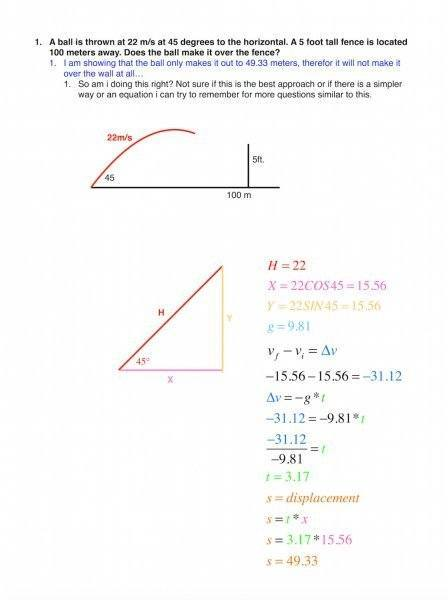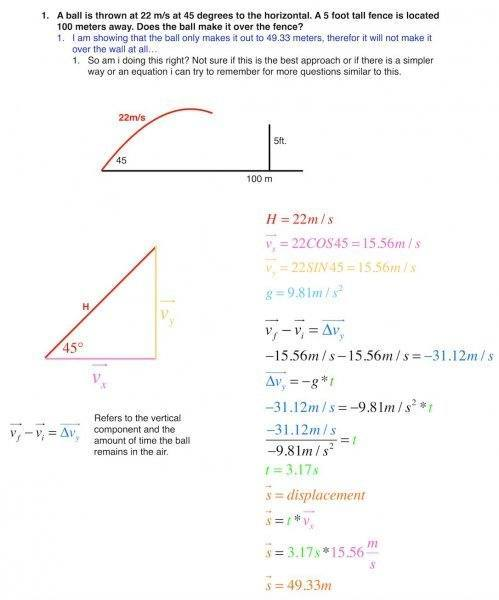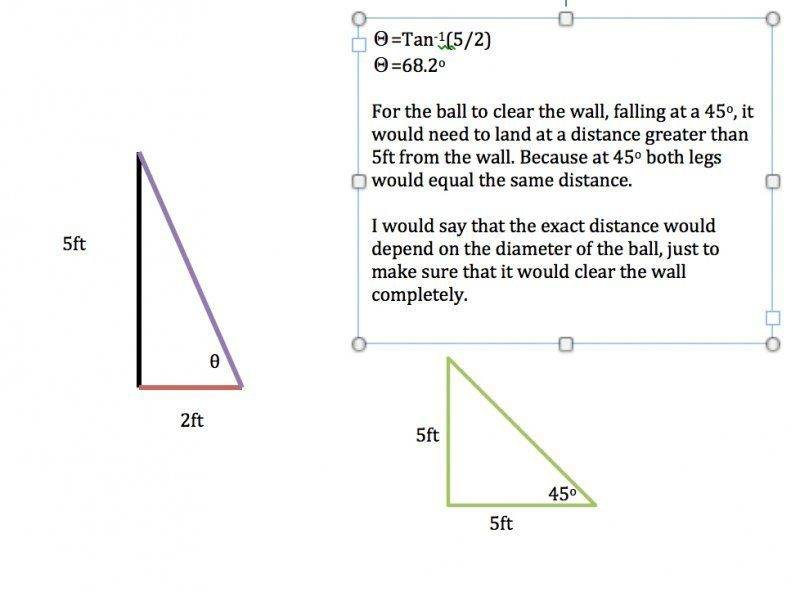# Will the ball clear the fence?

• keithcuda
In summary, the ball does not clear the fence because the distance traveled is not greater than the distance to the fence.

## Homework Statement

1. A ball is thrown at 22 m/s at 45 degrees to the horizontal. A 5 foot tall fence is located 100 meters away. Does the ball make it over the fence?

## Homework Equations## The Attempt at a Solution

You've written some numbers down, but whether they mean anything is another story.

What's the deal with vf - vi = -31.12? [You need to show units in your calculations always.] What does this even mean, in terms of the travel of the ball?

keithcuda said:

## Homework Statement

1. A ball is thrown at 22 m/s at 45 degrees to the horizontal. A 5 foot tall fence is located 100 meters away. Does the ball make it over the fence?

## Homework Equations

View attachment 77761

## The Attempt at a Solution

The first three equations are correct. You found the initial velocities in the x and y directions.

The motions in the x and y directions are independent. You can write a very simple equation for the motion in the x direction: x = x_0 + v_x * t (constant velocity, no acceleration). You can write a second equation for the motion in the y-direction. For this one, there is acceleration. [Be careful with the signs on the initial velocity and acceleration, here].

See: https://www.physicsforums.com/showpost.php?p=905663&postcount=2 for the equations for motion in one-dimension.

For the condition: "Ball over the fence", how would you define this with particular values for x and y?

Last edited:
Perhaps start with a description of how you think it might be solved first rather than just writing down equations. The equations might be relevant but best figure out the approach to take first.

SteamKing said:
You've written some numbers down, but whether they mean anything is another story.

What's the deal with vf - vi = -31.12? [You need to show units in your calculations always.] What does this even mean, in terms of the travel of the ball?

Here is my image and drawing with all of the units shown.. Sorry for not including them earlier.
https://www.physicsforums.com/attachments/77770Last edited:
Who's throwing? Randy Johnson? Or Warwick Davis?

Bystander said:
Who's throwing? Randy Johnson? Or Warwick Davis?
Roy Hobbs ;)

keithcuda said:
Here is my image and drawing with all of the units shown.. Sorry for not including them earlier.
https://www.physicsforums.com/attachments/77770

keithcuda said:
Here is my image and drawing with all of the units shown.. Sorry for not including them earlier.
https://www.physicsforums.com/attachments/77770View attachment 77771

Ok, I understand what you are doing now.

On the line with Delta v, you are stating that at the point that the ball impacts the ground, the downward velocity is the same magnitude as the upward velocity at the launch, but with opposite direction. You use the calculated Delta v and g to calculate the time aloft, and you use the horizontal component of the velocity times the time aloft to calculate the distance traveled. You note that since the distance traveled (49 m) < 100 m, the ball hits the dirt in front of the wall.

This looks good to me.

I would suggest adding some words to describe the equations -- to show that you know what you are doing. If you screw up the calculation (arithmetic error) but have the correct physics, your professor might give you some partial credit...

Quantum Defect said:
Ok, I understand what you are doing now.

On the line with Delta v, you are stating that at the point that the ball impacts the ground, the downward velocity is the same magnitude as the upward velocity at the launch, but with opposite direction. You use the calculated Delta v and g to calculate the time aloft, and you use the horizontal component of the velocity times the time aloft to calculate the distance traveled. You note that since the distance traveled (49 m) < 100 m, the ball hits the dirt in front of the wall.

This looks good to me.

I would suggest adding some words to describe the equations -- to show that you know what you are doing. If you screw up the calculation (arithmetic error) but have the correct physics, your professor might give you some partial credit...

Sorry I wasn't as clear earlier. It is hard for me to figure out what exactly I need to find and when. I found an example to my problem on Khanacademy.org and just watched it a few times and then used what i gained from the video to solve my problem. I was wanting to double check my approach and see if there was a different way to solve this or if the way i went about it is how it would be normally solved.

Cheers
Keith

How high can that ball bounce?TSny said:
How high can that ball bounce?OH boy! Ummm...Playing the devil... Suppose the distance traveled had worked out at 102m. Would the ball have cleared the wall?

CWatters said:
Playing the devil... Suppose the distance traveled had worked out at 102m. Would the ball have cleared the wall?

Sorry it took me a little while to get back, been working. I don't get weekends off very muchThe ball was thrown at a 45 degree angle. So it would also fall at a 45 degree angle.